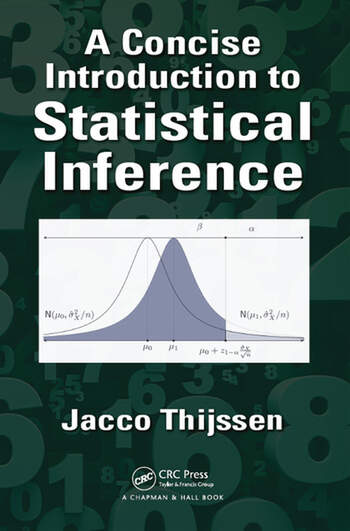## a concise introduction to statistical inference [book review]

[Just to warn readers and avoid emails about Xi’an plagiarising Christian!, this book was sent to me by CRC Press for a review. To be published in CHANCE.]This is an introduction to statistical inference. And with 180 pages, it indeed is concise! I could actually stop the review at this point as a concise review of a concise introduction to statistical inference, as I do not find much originality in this introduction, intended for “mathematically sophisticated first-time student of statistics”. Although sophistication is in the eye of the sophist, of course, as this book has margin symbols in the guise of integrals to warn of section using “differential or integral calculus” and a remark that the book is still accessible without calculus… (Integral calculus as in Riemann integrals, not Lebesgue integrals, mind you!) It even includes appendices with the Greek alphabet, summation notations, and exponential/logarithms.

“In statistics we often bypass the probability model altogether and simply specify the random variable directly. In fact, there is a result (that we won’t cover in detail) that tells us that, for any random variable, we can find an appropriate probability model.” (p.17)

Given its limited mathematical requirements, the book does not get very far in the probabilistic background of statistics methods, which makes the corresponding chapter not particularly helpful as opposed to a prerequisite on probability basics. Since not much can be proven without “all that complicated stuff about for any ε>0” (p.29). And makes defining correctly notions like the Central Limit Theorem impossible. For instance, Chebychev’s inequality comes within a list of admitted results. There is no major mistake in the chapter, even though mentioning that two correlated Normal variables are jointly Normal (p.27) is inexact.

“The power of a test is the probability that you do not reject a null that is in fact correct.” (p.120)

Most of the book follows the same pattern as other textbooks at that level, covering inference on a mean and a probability, confidence intervals, hypothesis testing, p-values, and linear regression. With some words of caution about the interpretation of p-values. (And the unfortunate inversion of the interpretation of power above.) Even mentioning the Cult [of Significance] I reviewed a while ago.

Given all that, the final chapter comes as a surprise, being about Bayesian inference! Which should make me rejoice, obviously, but I remain skeptical of introducing the concept to readers with so little mathematical background. And hence a very shaky understanding of a notion like conditional distributions. (Which reminds me of repeated occurrences on X validated when newcomers hope to bypass textbooks and courses to grasp the meaning of posteriors and such. Like when asking why Bayes Theorem does not apply for expectations.) I can feel the enthusiasm of the author for this perspective and it may diffuse to some readers, but apart from being aware of the approach, I wonder how much they carry away from this brief (decent) exposure. The chapter borrows from Lee (2012, 4th edition) and from Berger (1985) for the decision-theoretic part. The limitations of the exercise are shown for hypothesis testing (or comparison) by the need to restrict the parameter space to two possible values. And for decision making. Similarly, introducing improper priors and the likelihood principle [distinguished there from the law of likelihood] is likely to get over the head of most readers and clashes with the level of the previous chapters. (And I do not think this is the most efficient way to argue in favour of a Bayesian approach to the problem of statistical inference: I have now dropped all references to the likelihood principle from my lectures. Not because of the controversy, but simply because the students do not get it.) By the end of the chapter, it is unclear a neophyte would be able to spell out how one could specify a prior for one of the problems processed in the earlier chapters. The appendix on de Finetti’s formalism on personal probabilities is very much unlikely to help in this regard. While it sounds so far beyond the level of the remainder of the book.

This site uses Akismet to reduce spam. Learn how your comment data is processed.